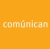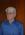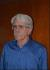# tanto por uno en peso de

## English translation: decimal fraction by weight of

GLOSSARY ENTRY (DERIVED FROM QUESTION BELOW)
 Spanish term or phrase: tanto por uno en peso de English translation: decimal fraction by weight of Entered by:

 14:14 Aug 5, 2019
Spanish to English translations [PRO]
Tech/Engineering - Environment & Ecology / Landfills
 Spanish term or phrase: tanto por uno en peso de I need some specialist help with getting the wording right for this formula, please. It is to do with the dissolved organic carbon (DOC) found in waste. Many thanks, as always. DOC: fracción de carbono orgánico biodegradable en el residuo: DOC=0,4∙A+0,17∙B+0,15∙C+0,3∙D A: tanto por uno en peso de la fracción de papel y textiles B: tanto por uno en peso de la fracción de resto de podas de jardín y bosques C: tanto por uno en peso de la fracción de restos de alimentos D: tanto por uno en peso de la fracción de madera
 ComunicanUnited KingdomLocal time: 06:45
 decimal fraction by weight of Explanation:Decimal fraction is numerically identical with percentage, except the decimal point is moved two places. Decimal fraction is the amount of a component of something, divided by the amount of the whole something. It is often computed by weight, but is sometimes computed by volume as well. In our case, for example, for A, the weight of the paper & textiles in the residue divided by the total weight of the residue: decimal fraction A = (paper & textiles)/(total weight). The percentage uses the computation of the decimal fraction as the first step, then multiplies by 100: A expressed as a percentage = [(paper & textiles)/(total weight)] X 100
Selected response from:

TomWalker
United States
Local time: 22:45
 Many thanks Tom (and everyone else who helped)4 KudoZ points were awarded for this answer

5decimal fraction by weight of
 TomWalker4weight parts per unit
 MPGSDiscussion entries: 3

29 mins   confidence:weight parts per unit

Explanation:
https://www.proz.com/search/

 MPGSLocal time: 07:45Works in fieldNative speaker of:SpanishPRO pts in category: 89 hrs   confidence:decimal fraction by weight of

Explanation:
Decimal fraction is numerically identical with percentage, except the decimal point is moved two places. Decimal fraction is the amount of a component of something, divided by the amount of the whole something. It is often computed by weight, but is sometimes computed by volume as well. In our case, for example, for A, the weight of the paper & textiles in the residue divided by the total weight of the residue:
decimal fraction A = (paper & textiles)/(total weight).
The percentage uses the computation of the decimal fraction as the first step, then multiplies by 100:
A expressed as a percentage = [(paper & textiles)/(total weight)] X 100

 TomWalkerUnited StatesLocal time: 22:45Specializes in fieldNative speaker of:EnglishPRO pts in category: 8Many thanks Tom (and everyone else who helped)

Login or register (free and only takes a few minutes) to participate in this question.

You will also have access to many other tools and opportunities designed for those who have language-related jobs (or are passionate about them). Participation is free and the site has a strict confidentiality policy.

## KudoZ™ translation help

The KudoZ network provides a framework for translators and others to assist each other with translations or explanations of terms and short phrases.